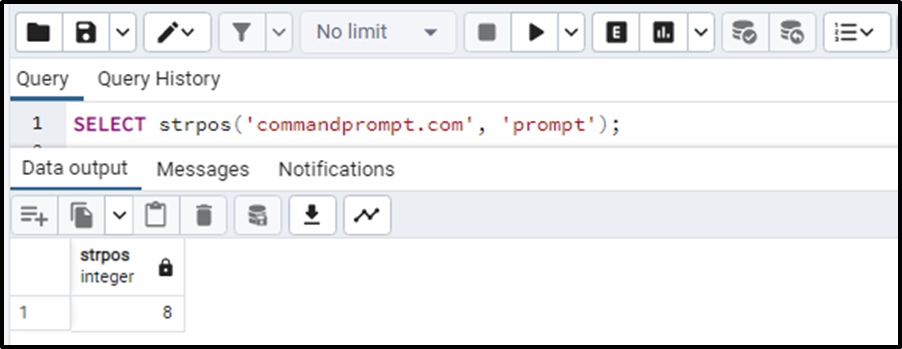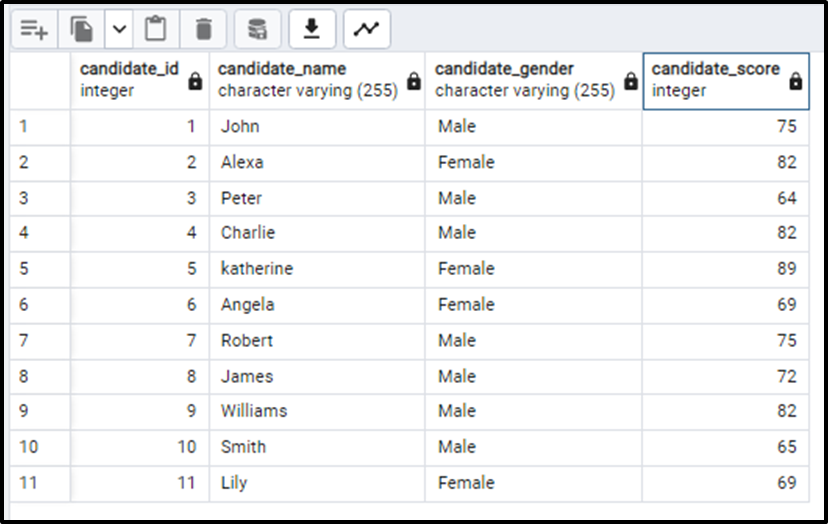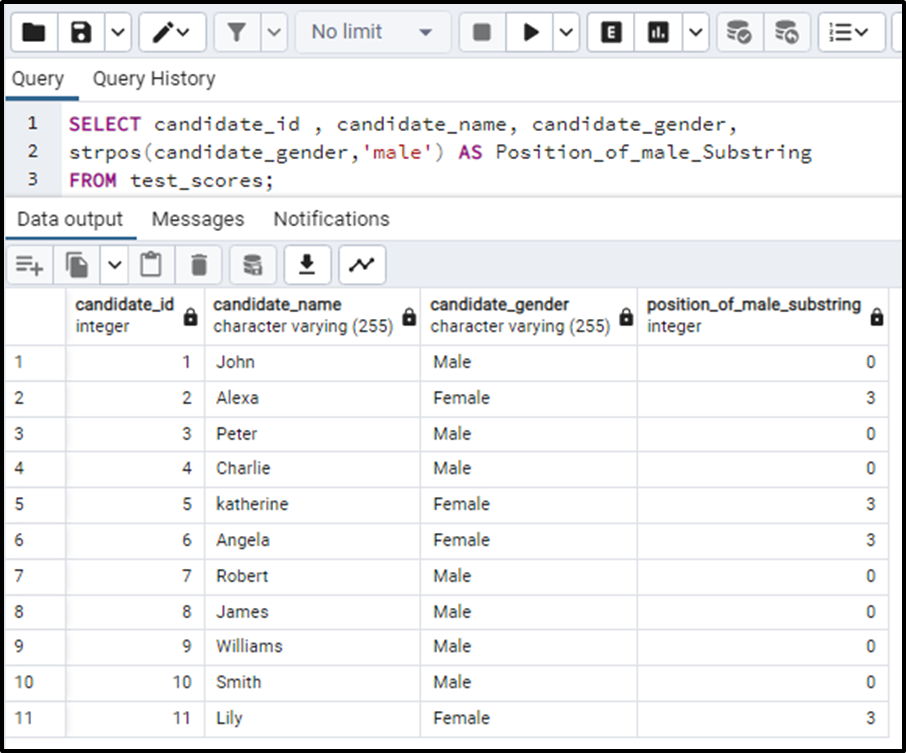##### How to Use strpos() Function in PostgreSQL

PostgreSQL offers many functions that can manipulate the string data or can be used to get some sort of information about that string. The strpos() function finds the position of a sub-string in a main-string. This function takes the main-string and the sub-string as arguments and returns the starting index of the position where the sub-string has occurred. Let’s dive deep into the workings of this function.

## How to Use strpos() Function in PostgreSQL?

The strpos() function determines the position of a sub-string or maybe a character in a main-string. Both the strings to be matched are given to the function as parameters. The basic syntax of this function is given below:

`strpos(Main_String, Sub_String);`

In the above syntax,

• The main string is the string in which the function will match the sub-string and will return the index of that sub-string.
• The second argument is the sub-string which we have to look for in the main-string.

The function gives the starting index of the sub-string occurrence in the main string. It means that the return data type of this function is INTEGER.

If in case the sub-string is missing in the main string, the function will return 0. And if any of the parameters of the function are NULL the function will also return NULL.

Note: This function works similarly to the position() function. Just the positioning of arguments and the syntax are a little different.

Let’s consider the examples so that we can have a better idea of this function.

Example

To illustrate the concept of the strpos() function, consider the following query:

`SELECT strpos('commandprompt.com', 'prompt');`

The above query will return the starting index of the “prompt” in the main string like this:We can clearly see that the “prompt” starts from index 8 in the main string.

We can also apply this function to the table. Let’s consider a table “test_scores” having 4 columns; candidate_id , candidate_name, candidate_gender and candidate_score.Now we will find the position of sub-string “male” in the column candidate_gender. The query will be written as:

```SELECT candidate_id , candidate_name, candidate_gender,
strpos(candidate_gender,'male') AS Position_of_male_Substring
FROM test_scores;```

The output of the above query will give a separate column for determining the position of “male” in the candidate_gender column. The output looks like this:Here we can see that the function has returned the position of the “male” sub-string in the gender column. For the entries with the value “Male,” the strpos() function returns 0 because the function does case-sensitive matching and the function was not able to find the ”male” with lower case. While the sub-string “male” can be found in the string “Female”. so, all those entries where the value of gender is female get the value of 3.

So in this article, we have seen how strpos() function functions on a single main string and also on the column of a table. So this was all about this strpos() function.

Conclusion

The strpos() function in PostgreSQL is used to determine the position/location of a sub-string in a main string. The function returns an integer that specifies the first index of the occurrence of the specified sub-string the main string. If the sub-string is missing from the string, simply a 0 will be returned as seen in the above example. Also, remember that the function does case-sensitive matching.